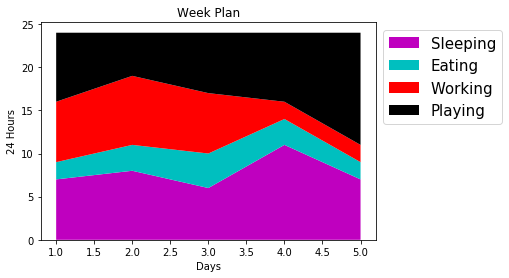Breaking News
Home / Data Science / Stack Plot using Matplotlib# Stack Plot using Matplotlib

6 Stack Plot

### Plot a Stack Plot using Matplotlib¶

Stack Plots are used to visualize multiple linear plots, stacked on top of each other. With a regular line plot, you’d plot the relationship between X and Y. Here, we’re plotting multiple Y features on a shared X-axis, one on top of the other:

In :
import matplotlib.pyplot as plt
days = [1,2,3,4,5]
sleeping = [7,8,6,11,7]
eating = [2,3,4,3,2]
working = [7,8,7,2,2]
playing = [8,5,7,8,13]
Lab = ['Sleeping','Eating','Working','Playing']
plt.title('Week Plan')
plt.xlabel('Days')
plt.ylabel('24 Hours')
plt.stackplot(days,sleeping,eating,working,playing,labels=Lab,colors=['m','c','r','k'])
plt.legend(fontsize=15,bbox_to_anchor=(1.4,1.0))
plt.show()